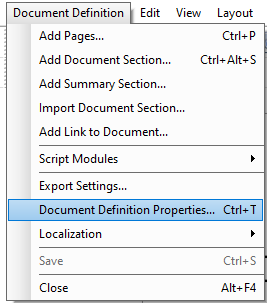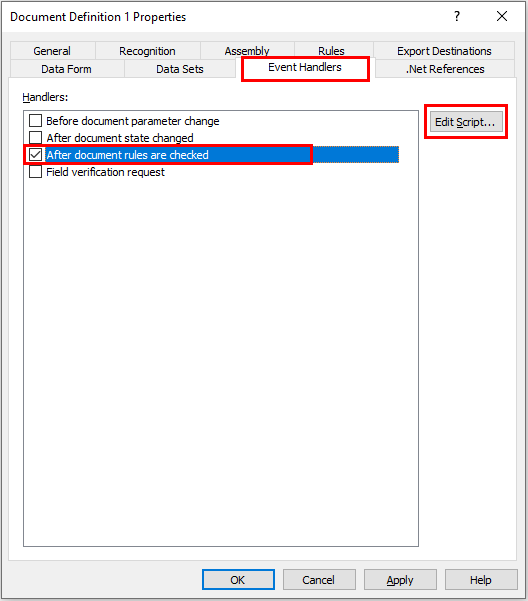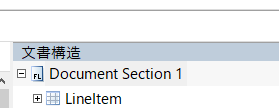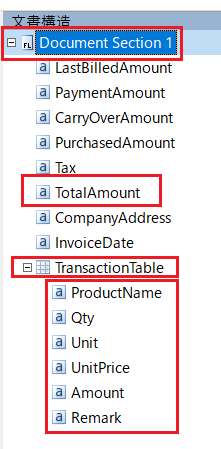## Working with the elements (rows, columns) of a Table or Repeating group in scripts

There are cases when the user wishes to add some additional configuration to each element of the Table or Repeating group. Please follow the below steps of a basic method of doing that.

1. Open "Document Definition Properties" in Document Definition editor:2. Go to the "Event Handlers" tab, choose the "After document rules are checked" and click the "Edit Script" button.3. Get the number of rows of the Table of Repeating group. Use the following code snippet:
`int rowCount = Document.Field( <Table or Repeating Group full path> ).Items.Count;`
4. Example of usage if the document structure is as shown below:1. Getting the number of rows in a table:

`int rowCount = Document.Field( "Document Section 1\\LineItem" ).Items.Count;`
2. If it is problematic to write the path many times, you can write the script like this:

`string docSec01 = "Document Section 1";IField lineItem = Document.Field( docSec01 + "\\LineItem" );int rowCount = lineItem.Items.Count;`
3. Class: IField is ABBYY FlexiCapture's own class that stores instances of document-defined fields. You can perform various operations on the instance according to the specifications. See ABBYY Online Help article for details.

5. Example of accessing each row and column of the Table or Repeating Group if the document structure is as shown below:1. The script example (C#):
`string productName = "";string qty = "";string unit = "";string unitPrice = "";string amount = "";`
2. Accessing the value of an element if it is not a Table or Repeating group element:

`string totalAmount = Document.Field("Document Section 1\\TotalAmount").Text;`
3. Accessing the value of an element if it is a Table or Repeating group element:

`IField table = Document.Field("Document Section 1\\TransactionTable");`
4. Getting the number of rows:
`int rowCount = table.Items.Count;`
5. Accessing string values for column elements in each row of a Table/Repeating group:
`for( int i=0 ; i<rowCount ; i++ ){    productName = table.Items[i].Field("ProductName").Text;    qty = table.Items[i].Field("Qty").Text;    unit = table.Items[i].Field("Unit").Text;    unitPrice = table.Items[i].Field("UnitPrice").Text;    amount = table.Items[i].Field("Amount").Text;}`

Have more questions? Submit a request

1 comment

•